CBSE Sample Papers for Class 12 Computer Science Paper 3 are part of CBSE Sample Papers for Class 12 Computer Science. Here we have given CBSE Sample Papers for Class 12 Computer Science Paper 3.

## CBSE Sample Papers for Class 12 Computer Science Paper 3

 Board CBSE Class XII Subject Computer Science Sample Paper Set Paper 3 Category CBSE Sample Papers

Students who are going to appear for CBSE Class 12 Examinations are advised to practice the CBSE sample papers given here which is designed as per the latest Syllabus and marking scheme, as prescribed by the CBSE, is given here. Paper 3 of Solved CBSE Sample Paper for Class 12 Computer Science is given below with free PDF download Answers.

Time: 3 Hours
Maximum Marks: 70

General Instructions:

• All questions are compulsory within each Section.
• Programming Language in SECTION A : C++.

SECTION A

Question 1.
(a) Out of the following find those identifiers, which can be used for naming variable, constant or function in a C++ program:
throw, Num2, sum^3, THIS, Float, return, _Sum, first name
(b) Which C++ header file(s) will be essentially required to be included to run/execute the following C++ code?

void main()
{
char Info[]="Indian Army";
for(int I=5; I<strlen(Info): I++)
puts(Info):
}

(c) Rewrite the following program after removing the syntactical error(s) (if any). Underline each correction.
Note: Assume all required header files are already being included in the program.

Main()
{
Float x, y, z;
cout<<"Enter two numbers": cin>>a>>b
cout<<"The numbers in reverse order are"<<b, a;
}

(d) Find and write the output of the following program:
Note: Assume all required header files are already being included in the program.

struct Play
{
char Arr;
int n;
};
void main()
{
Play P = {"Magic",500};
P.Arr = 'Q';
P.Arr = 'L' ;
P.n+= 50;
cout<<P.Arr<<P.n<<endl;
Play R = P;
R.n- = 120;
cout<<R.n<<endl;
}

(e) Observe the following C+ + code carefully and obtain the output, which will appear on the screen after the execution of it.
Note: Assume all required header files are already being included in the program.

class Array
{
int num;
int size:
public:
void Change(int num, int arr[], int size)
{
for(int n=0; n<size; n++)
if (n<num)
arr[n]+ = n;
else
arr[n] * = n;
}
void display(int arr[], int size)
{
for(int n=0; n<size; n++)
(n%2!=0)? cout<<arr[n]<<"#":cout<<arr[n]<<endl:
}
};
void main()
{
clrscr();
Array a:
int array[] = {50,40,90,10,60,70};
a.Change(4, array, 7);
a.display(array, 7);
getch();
}

(f) The following code is from a game, which generates a set of 4 random numbers. Ravi is playing this game, help him to identify the correct option(s) out of the four choices given below as the possible set of such numbers generated from the program code so that he wins the game. Also, find out the minimum and maximum value that can be assigned to the variable Number used in the code at the time when the value of I is 2.
Note:
Assume all required header files are already being included in the program.
random(n) function generates an integer between 0 and n-1

const int a = 15;
void main()
{
randomize();
int POINT = 5, Number;
for(int I=1; I<=4; I++)
{
Number = a+random(POINT);
cout<<Number<<":";
POINT--;
}
}

(i) 19: 16 : 15: 18 :
(ii) 14: 18: 15: 16 :
(iii) 19: 16 : 14: 18 :
(iv) 19: 16: 15: 16 :

Question 2.
(a) What do you understand by polymorphism? Give a suitable example.
(b) Answer the questions (i) and (ii) after going through the following program:

class Student
{
int stuID;
float fees;
public:
Student() //Function1
{
stuID=108;
fees=5400;
}
void Course(float C) //Function2
{
}
~Student() //Function3
{
cout<<"Course Cancelled"< }
Student(int S, char A[], float C) //Function4
{
stuID=S;
fees=C;
}
};
void main() //Line1
{ //Line2
Student S1, S2(102, "Meerut", 20); //Line3
for(int i=0; i<5; i++) //Line4
{ //Line5
S1 .Course(50); //Line6
S2.Course(45); / / Line7
} //Line8
} //Line9

(i) In object-oriented programming, what are Function1 and Function4 combined together as?
(ii) How many times the message “Course Cancelled” will be displayed after executing the above C++ code? Out of Line1 to Line9, which line is responsible to display the message “Course Cancelled”?
(c) Define a class Batsman with the following specifications:
Private members
bcode – 4 digit code (maybe number and character)
bname – 20 characters
innings, not out, runs – integer type
batavg – It is calculated according to the formula batavg= runs/(innings-not out)
calcavg() – Function to compute batavg
Public members
readdata() – Function to accept values of bcode, bname, innings, not out and invoke the function calcavg()
displaydata() – Function to display the data members on the screen.
(d) Answer the questions (i) to (iv) based on the following code:

class Shape
{
int length;
protected:
int width, height;
public:
void getDimension(int, int, int);
void dispDimension();
};
class SideShape:protected Shape
{
int SideLength, SideWidth;
protected:
void getSide(int, int);
public:
void dispSide();
};
class SubShape:public SideShape
{
int SubLength;
void display(void);
public:
void enter();
};

(i) Name of the base class and derived class of the class SlideShare.
(ii) Name of the data member(s) that can be accessed from function dispSide().
(iii) Name of the private member function(s) of class subshape.
(iv) Which type of inheritance is illustrated in the above example?

Question 3.
(a) Write a code for a function Change (int a[ ], int size) in C++, to add 8 in all the odd values and 6 in all the even values of the array a.
e.g. If the array a is:
8, 7, 5, 4, 5, 2, 6
The output will be:
14, 15, 13, 10, 13, 8, 12
(b) An array X   is stored in memory with each element requiring 4 bytes of storage. If the base address of the array is 1000, calculate the location of X   when the array X is stored using column-major order.
(c) Write a function in C++ which accepts an integer array and its size as an argument and assign the elements in the 2-D array in the following format:
e.g. If the 1-D array is: 1, 2, 3, 4
The resultant 2-D array should bee.g. If the 1-D array is: 1, 2, 3
The resultant 2-D array should be(d) Evaluate the following postfix notation of expression
True, False, AND, True, True, NOT, OR, AND
(e) Each node of a stack contains the following information, in addition to pointer field
(i) EmpCode of employee
(ii) EName of employee
Give the structure of node for the linked stack in question. Top is a pointer that points to the topmost node of the stack. To push a node into the stack, which is dynamically allocated stack of items considering the following code is already written as a part of the program.

struct node
{
int EmpCode;
char Ename;
};
class Stack
{
node * Top;
public:
Stack()
{
Top=NULL;
}
void PUSH();
void POP();
~Stack();
};

Question 4.
(a) Observe the following code and answer the following question:

void main()
{
char ch = 'z';
fstream fileout("data.dat",ios::app);
fileout<<ch;
int p=fileout.tellg();
cout<<p;
}

What is the output if the file content before the execution of the program is the string “ARIHANT”?
Note: ” ” are not part of the file.
(b) Write a function COUNT_HOW() in C++ to count the presence of the word “How” in a text file “MEMO.TXT”.
e.g.
If the content of the file “MEMO.TXT” is as follows:
How are you?
Don’t go there
How will you go?
The function COUNT_HOW will display the following message:
Count of how in file 2
Note In the above example, how occurring as a part of word whose is not considered.
(c) Given a binary file “CUSTOMER.DAT, containing records of the following structure type

class Customer
{
char C_Name;
char Area;
char C_Phone_No;
public:
void Account();
void Disp();
int CheckCode(char AC [])
{
return strcmp(Area, AC);
}
};

Write a function COPYFILE() in C++, that would copy only those records having Area as “SOUTH” from “CUSTOMER.DAT’ to “BACKUR.DAT.

SECTION B

Question 5.
(a) Observe the following EMPLOYEE and MANAGER tables carefully and write the name of the RDBMS operation which will be used to produce the output as shown in RESULT. Also, find the Degree and Cardinality of the RESULT.(b) Write SQL queries for (i) to (iv) and find outputs for SQL queries (v) to (viii), which are based on the tables.(i) To display the name of all sports with their SCode.
(ii) To display details of those sports which are having PrizeMoney more than 9000.
(iii) To display the content of the SPORTS table in ascending order of ScheduleDate.
(iv) To display the sum of PrizeMoney for each of the Number of participation groupings (as shown in the column, Number 2 or 4).

(v) SELECT COUNT(DISTINCT Number) FROM SPORTS;
(vi) SELECT MAX(ScheduleDate). MIN(ScheduleDate) FROM SPORTS;
(vii) SELECT A.SportName, A.PrizeMoney,
B.Name FROM SPORTS A, COACH B
WHERE A.SCode=B.SCode AND Name= 'Ravi';
(viii) SELECT SportName, Number, CCode, Name
FROM SPORTS, COACH
WHERE SPORTS.SCode=COACH SCODE AND PrizeMoney>20000;

Question 6.
(a) Using Boolean laws, prove that $$\bar { X } \bar { Y } +\bar { X } +XY=\bar { X } +Y$$
(b) Draw the digital circuit for the function.
$$F\left( X+Y \right) \cdot \left( \bar { X } +\bar { Z } \right) \cdot \left( Y+Z \right)$$
(c) Write the POS form of Boolean function F, which is represented in a truth table as follows: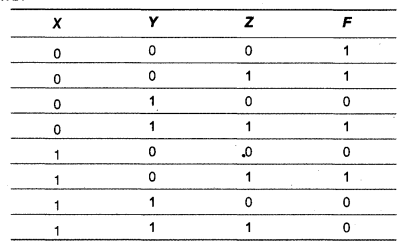(d) Reduce the following Boolean expression using K-map:
F(P, Q, R, S) = Π (0, 2, 3, 5, 6, 7, 8, 10, 11, 12, 15)

Question 7.
(a) What are the design goals of XML?
(b) How the firewall protects our network?
(c) How trojan horses are different from worms? Mention any one difference.
(d) Which network device is used to filter data traffic at a network boundary?
(e) DISTANCE EDUCATION is located in DELHI and is planning to go in for networking of 4 wings for better interaction. The details are given below: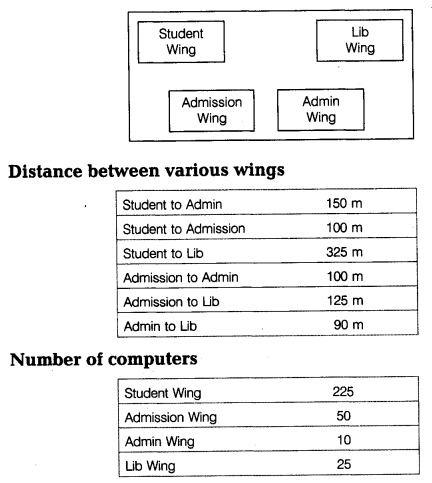(i) Suggest the most appropriate building to building cable layout to connect all three buildings for efficient communication.
(ii) Suggest the most suitable place (i.e. Wing) to house the server with a suitable reason.
(iii) Suggest the type of networking (LAN, MAN, WAN) for connecting Lib Wing to Admin Wing. Justify your answer.
(iv) The institute is planning to link its study center situated in Delhi. Suggest an economical way to connect it with reasonable high speed. Justify your answer.
(f) Categorize the following under Open Source based Software and Proprietary Software.
(ii) Apache
(iii) Skype
(iv) MySQL
How is an E-mail different from a chat?

(a) Identifiers which can be used for naming variable, constant or function in a C++ program are Num2, THIS, Float, _Sum
(b) The following two header files are required to execute the given code:

(i) → strlen()
(ii) → puts()

(c) void main()
{
float x, y, z;
cout<<"Enter two numbers": cin>>x>>y:
cout<<"The numbers in reverse order are"<<y<<x:
}

(d) Output
MaLiQ550
430
(e) Output
50
41 # 92
13 # 240
350 # 0
(f) (iv) 19 : 16: 15: 16: is the correct option.
When I = 2
The minimum value of variable Number can be 15
The maximum value of variable Number can be 18

(a) Polymorphism is the way of representing one form-multiple behaviour iq a programming language. Function overloading is one example of polymorphism, where more than one function carrying same name behave differently with different set of parameters passed to them.

void Show()
{
cout<<"Example"<<endl;
}
void Show(int a)
{
cout<<"Value of a "<<a;
}
void main()
{
Show();
Show(5);
}

(b) (i) Function 1 and Function4 combined together referred as constructor overloading, i.e. polymorphism.
(ii) 2 times the message “Course Cancelled” will be displayed. Line3 is responsible to display the message “Course Cancelled”.

(c) class Batsman
{
private:
char bcode;
char bname;
int innings, notout, runs;
float batavg;
void calcavg();
public:
void displaydata();
};
void Batsman::calcavg()
{
batavg = runs/(innings-notout);
}
{
cout<<"Enter the four digit code";
for(int i = 0; i < 4; i++) cin>>bcode[i];
cout<<"Enter name":
gets(bname);
cout<<"Enter innings": cin>>innings ;
cout<<"Enter notout": cin>>notout;
cout<<"Enter runs"; cin>>runs; calcavg():
}
void Batsman::displaydata()
{
cout<<"\nBatsman code:";
fort(int i=0; i<4; i++) .
cout<<bcode[i];
cout<<”\nBatsman Name:”;
puts(bname);
cout<C'\nInnings : "<<innings;
cout<<"\nNotouts:"<<notout;
cout<<"\nRuns:"<<runs;
cout<<"\nThe Average:"<<batavg;
}

(d) (i) Base class of SideShape : Shape
Derived class of SideShape : SubShape
(ii) SideLength, SideWidth, width, height.
(iii) display()
(iv) Multi Level Inheritance

(a) void Change(int a[], int size)
{
for(int i=0; i<size; i++)
{
if(a[i]%2==0)
a[i]+=6;
else,
a[i]+=8;
}
}

(b) Base address B = 1000
W = 4 bytes
N = 20,
M = 10
L1= 0,
L2 = 0
I = 5,
J = 15
X[I][J] = B + W * [{I-L1) + M*(J – L2)]
X = 1000 + 4 * [(5 – 0) + 10 * (15 – 0)]
= 1000 + 4 *(5 + 150)
= 1000 + 4 * 155 = 1000 + 620
= 1620

(c) void convertdnt a[],int n) //a[] is the 1-D array
{
int an, i, j, k = 0, 1; //a[][] is the resultant 2-D array.
for(i =0; i < n ; i++)
{
k = n - i;
l = 0;
for(j=0; j<n; j++)
{
if (l! = k)
{
an[i][j] = a[l];
l++;
}
else if (l = k && j ! = n)
an[i ][j] = 0;
}
}
for(i = 0; i < n; i ++)
{
cout<<"\n";
for(j = 0; j < n; j++)
cout<<an[i][j]<<"\t";
}
}

(d) True, False, AND, True, True, NOT, OR, AND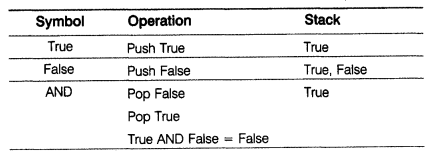Result: False

(e) struct node
{
int EmpCode;
char EName;
};
void PUSH(node *T0P, int code, char nm[])
{
node *temp;
temp=new node;
temp->EmpCode=code;
strcpy(temp->EName,nm);
if (TOP == NULL)
TOP=temp;
else
{
TOP=temp;
}
}

(a) 8

(b) void COUNT_HOW()
{
fstream File;
File.open("MEMO.TXT", ios::in);
char word:
int c = 0;
File>>word:
while(!File.eof())
{
for(int i=0; i<strlen(word); i++) word[i] = tolower(word[i]); if(strcmp(word, "how")==0) C++; File>>word;
}
cout<<"Count of how in file "<<c<9000;
(iii) SELECT * FROM SPORTS ORDER BY ScheduleDate;
(iv) SELECT SUM(Prize Money), Number FROM SPORTS GROUP BY Number;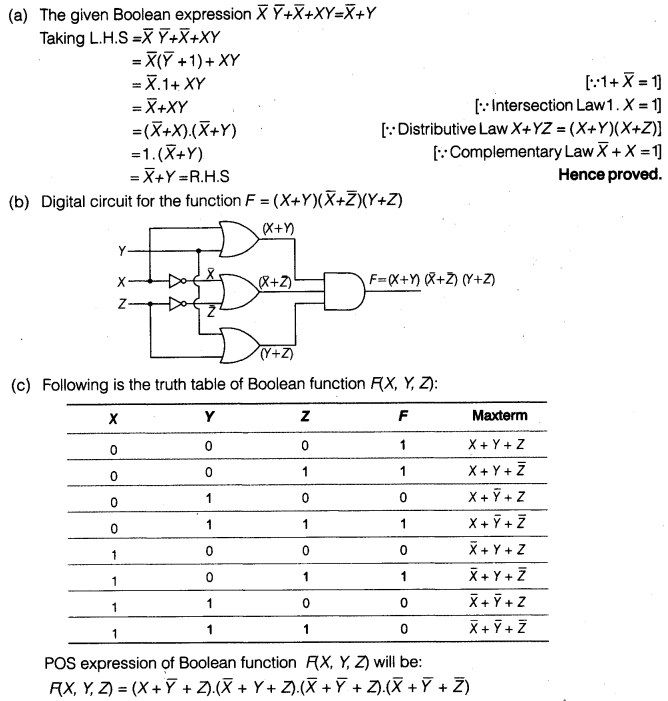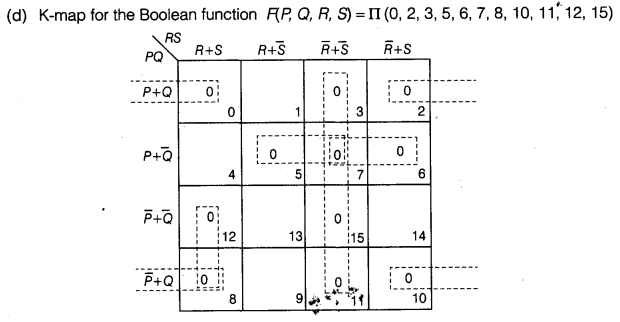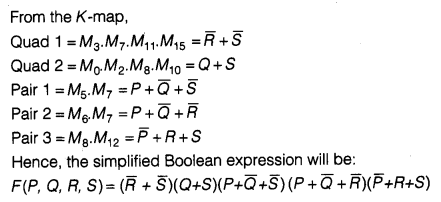(a) The design goals of XML emphasize simplicity, generality, and usability over the Internet.
(b) A firewall is a part of a computer system or network that is designed to block unauthorized access while permitting authorized communications. It is a device or set of devices configured to permit, deny, encrypt, decrypt or proxy all computer traffic between different security domains based upon a set of rules and other criteria.
(c) Difference between trojan horses and worms is as follows:

 Trojan Horses Worms It is a code hidden in a program that looks safe to run but in background performs malicious actions. Thus, it requires the intervention of users. It is a self-replicating computer program that works and propagates itself without the use of another program or action or intervention by the user.

(d) Bridge
(e) (i) Cable Layout(ii) Since the maximum number of computers are in the Student Wing. So, a suitable place to house the server is Student Wing.
(iii) Since the difference between Lib Wing and Admin Wing is small, so the type of networking is small, i.e. LAN.
(iv) Broadband connection as it is quite economical and speedy.
(f) Open Source based Software